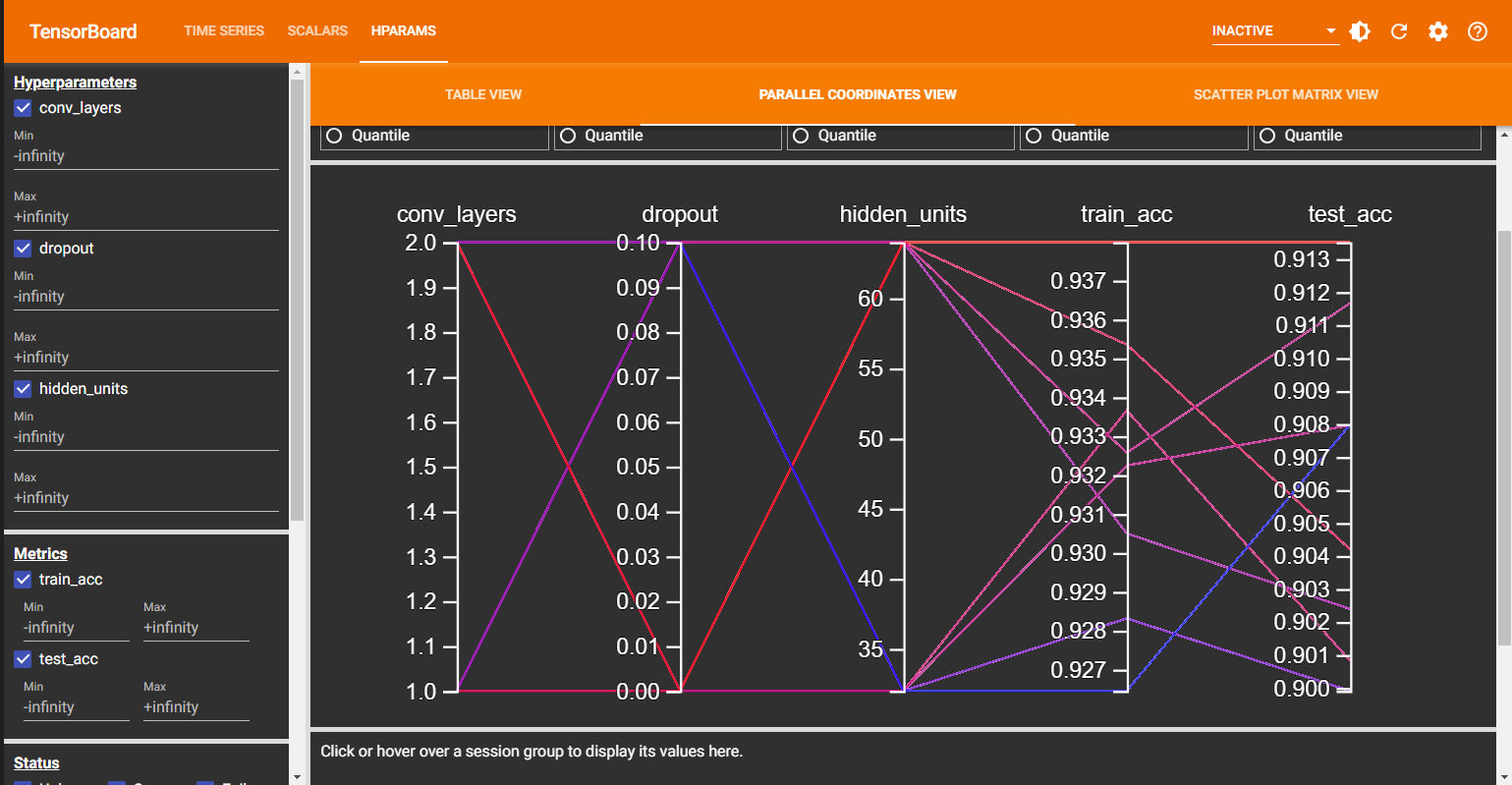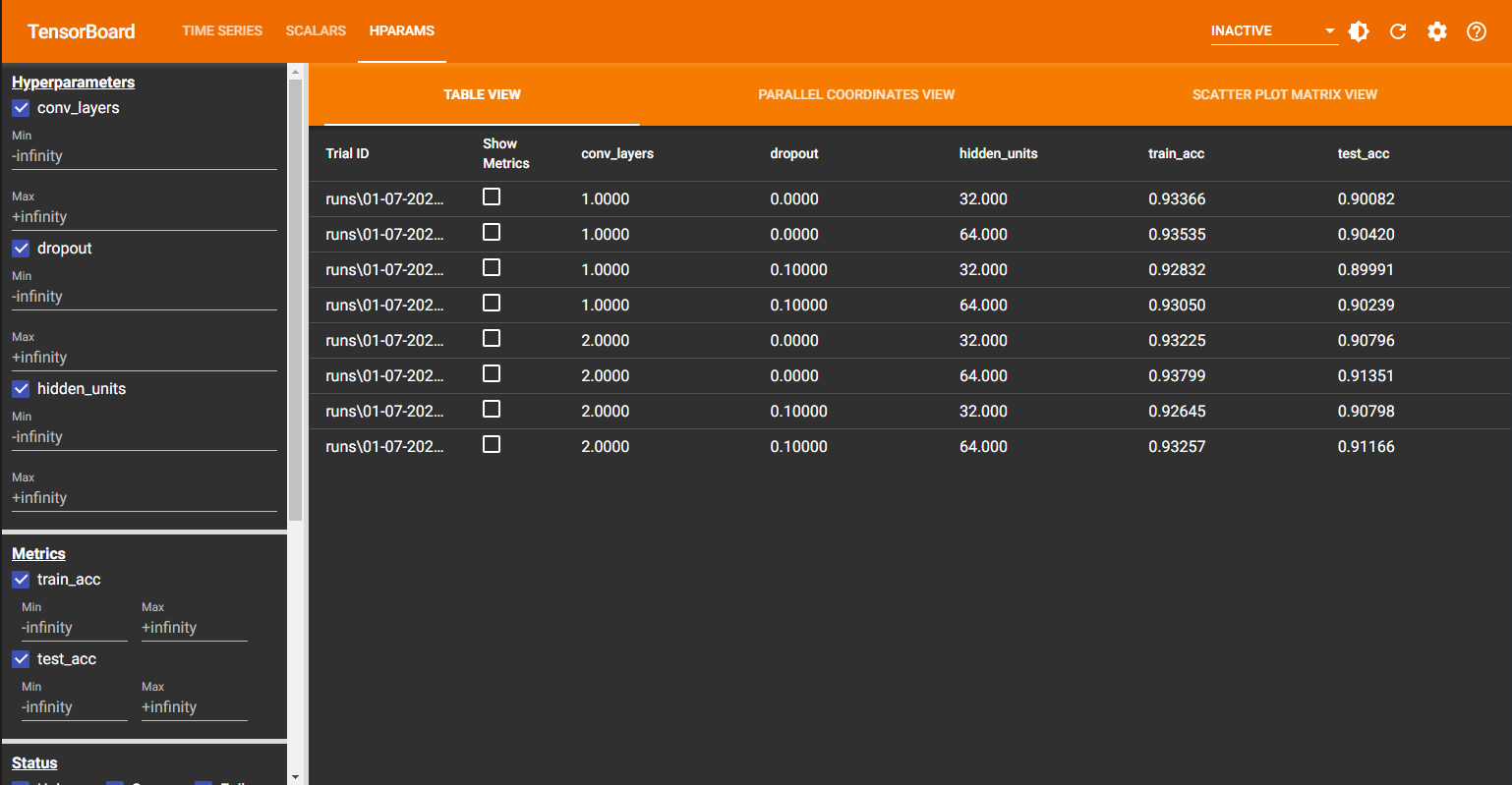## Experiment Tracking and Hyperparameter Tuning with TensorBoard in PyTorch 🔥

1 Jul 2023## Introduction

Tracking Experiments and Tuning Hyperparameters with TensorBoard in PyTorch

Experiment tracking involves logging and monitoring machine learning experiment data, and TensorBoard is a useful tool for visualizing and analyzing this data. It helps researchers understand experiment behavior, compare models, and make informed decisions.

Hyperparameter tuning is the process of finding the best values for configuration settings that impact model learning. Examples include learning rate, batch size, and number of hidden layers. Appropriate tuning improves model performance and generalization.

Hyperparameter tuning strategies include manual search, grid search, random search, Bayesian optimization, and automated techniques. These methods systematically explore and evaluate different hyperparameter values.

Using evaluation metrics like accuracy or mean squared error, you can assess model performance during tuning. Effective hyperparameter tuning leads to improved model results on unseen data.

In this blog, we’ll demonstrate hyperparameter tuning using grid search with the FashionMNIST dataset and a custom VGG model. Stay tuned for future blogs on other tuning algorithms.

Let’s begin!## Install and Import Dependencies

Start by opening a new python notebook on Jupyter or on Google Colab. Write these commands in code block to install and import the dependencies.

%pip install -q torchinfo torchmetrics tensorboard

import torch
import torchvision
import os
from torchvision.transforms import Resize, Compose, ToTensor
import matplotlib.pyplot as plt
from torchinfo import summary
import torchmetrics
from tqdm.auto import tqdm
from torch.utils.tensorboard import SummaryWriter


BATCH_SIZE = 64

if not os.path.exists("data"): os.mkdir("data")

train_transform = Compose([Resize((64,64)),
ToTensor()
])
test_transform = Compose([Resize((64,64)),
ToTensor()
])

training_dataset = torchvision.datasets.FashionMNIST(root = "data",
train = True,
transform = train_transform)

test_dataset = torchvision.datasets.FashionMNIST(root = "data",
train = False,
transform = test_transform)

batch_size=BATCH_SIZE,
shuffle=True,
)

batch_size = BATCH_SIZE,
shuffle = False,
)
• Here, we initiate a batch size of 64. Generally, you would want to go for the maximum batch size that your GPU can handle without giving the cuda out of memory error.
• We define the transforms to convert our images to Tensors.
• We initiate the training datasets and the test datasets from the built-in FashionMNIST dataset in torchvision datasets. We set the root folder as the data folder, download as True because we want to download the dataset and train as True for the training data and False for the test data.
• Next, we define the training and test dataloaders.

We can see how many images we have in our training and testing dataset using this command.

print(f"Number of Images in test dataset is {len(test_dataset)}")
print(f"Number of Images in training dataset is {len(training_dataset)}")

Number of Images in test dataset is 10000
Number of Images in training dataset is 60000

## Create a TinyVGG Model

I am demonstrating experiment tracking using this custom model. But you can use any model of your choice.

class TinyVGG(nn.Module):
"""
A small VGG-like network for image classification.

Args:
in_channels (int): The number of input channels.
n_classes (int): The number of output classes.
hidden_units (int): The number of hidden units in each convolutional block.
n_conv_blocks (int): The number of convolutional blocks.
dropout (float): The dropout rate.
"""

def __init__(self, in_channels, n_classes, hidden_units, n_conv_blocks, dropout):
super().__init__()
self.in_channels = in_channels
self.out_features = n_classes
self.dropout = dropout
self.hidden_units = hidden_units

# Input block
self.input_block = nn.Sequential(
nn.Dropout(dropout),
nn.ReLU(),
)

# Convolutional blocks
self.conv_blocks = nn.ModuleList([
nn.Sequential(
nn.Dropout(dropout),
nn.ReLU(),
nn.MaxPool2d(kernel_size=2, stride=2),
) for _ in range(n_conv_blocks)
])

# Classifier
self.classifier = nn.Sequential(
nn.Flatten(),
nn.LazyLinear(out_features=256),
nn.Dropout(dropout),
nn.Linear(in_features=256, out_features=64),
nn.Linear(in_features=64, out_features=n_classes),
)

def forward(self, x):
"""
Forward pass of the network.

Args:
x (torch.Tensor): The input tensor.

Returns:
torch.Tensor: The output tensor.
"""

x = self.input_block(x)
for conv_block in self.conv_blocks:
x = conv_block(x)
x = self.classifier(x)
return x

## Define Training and Test Functions


def train_step(dataloader, model, optimizer, criterion, device, train_acc_metric):
"""
Perform a single training step.

Args:
model (torch.nn.Module): The model to train.
optimizer (torch.optim.Optimizer): The optimizer for the model.
criterion (torch.nn.Module): The loss function for the model.
device (torch.device): The device to train the model on.
train_acc_metric (torchmetrics.Accuracy): The accuracy metric for the model.

Returns:
The accuracy of the model on the training data.
"""

# Move the data to the device.
X = X.to(device)
y = y.to(device)

# Forward pass.
y_preds = model(X)

# Calculate the loss.
loss = criterion(y_preds, y)

# Calculate the accuracy.
train_acc_metric.update(y_preds, y)

# Backpropagate the loss.
loss.backward()

# Update the parameters.
optimizer.step()

return train_acc_metric.compute()

def test_step(dataloader, model, device, test_acc_metric):
"""
Perform a single test step.

Args:
model (torch.nn.Module): The model to test.
device (torch.device): The device to test the model on.
test_acc_metric (torchmetrics.Accuracy): The accuracy metric for the model.

Returns:
The accuracy of the model on the test data.
"""

# Move the data to the device.
X = X.to(device)
y = y.to(device)

# Forward pass.
y_preds = model(X)

# Calculate the accuracy.
test_acc_metric.update(y_preds, y)

return test_acc_metric.compute()


## TensorBoard Summary Writer

def create_writer(
experiment_name: str, model_name: str, conv_layers, dropout, hidden_units
) -> SummaryWriter:
"""
Create a SummaryWriter object for logging the training and test results.

Args:
experiment_name (str): The name of the experiment.
model_name (str): The name of the model.
conv_layers (int): The number of convolutional layers in the model.
dropout (float): The dropout rate used in the model.
hidden_units (int): The number of hidden units in the model.

Returns:
SummaryWriter: The SummaryWriter object.
"""

timestamp = str(datetime.now().strftime("%d-%m-%Y_%H-%M-%S"))
log_dir = os.path.join(
"runs",
timestamp,
experiment_name,
model_name,
f"{conv_layers}",
f"{dropout}",
f"{hidden_units}",
).replace("\\", "/")
return SummaryWriter(log_dir=log_dir)

## Hyper Parameter Tuning

Here, there are several hyperparameters as you can see - Learning Rate, Number of Epochs, type of optimizer, number of convolution layers, dropout and number of hidden units. We can first fix the learning rate and number of epoch and try to find the best number of convolution layers, dropout and hidden units. Once we have those, we can then tune the number of epochs and learning rate.

We store the parameter values that we want to search over in the dictionary hparams_config

# Fixed Hyper Parameters/
EPOCHS = 10
LEARNING_RATE = 0.0007
"""
This code performs hyperparameter tuning for a TinyVGG model.

The hyperparameters that are tuned are the number of convolutional layers, the dropout rate, and the number of hidden units.

The results of the hyperparameter tuning are logged to a TensorBoard file.
"""

experiment_number = 0

# hyperparameters to tune
hparams_config = {
"n_conv_layers": [1, 2, 3],
"dropout": [0.0, 0.25, 0.5],
"hidden_units": [128, 256, 512],
}

for n_conv_layers in hparams_config["n_conv_layers"]:
for dropout in hparams_config["dropout"]:
for hidden_units in hparams_config["hidden_units"]:
experiment_number += 1
print(
f"\nTuning Hyper Parameters || Conv Layers: {n_conv_layers} || Dropout: {dropout} || Hidden Units: {hidden_units} \n"
)

# create the model
model = TinyVGG(
in_channels=1,
n_classes=len(training_dataset.classes),
hidden_units=hidden_units,
n_conv_blocks=n_conv_layers,
dropout=dropout,
).to(device)

# create the optimizer and loss function
criterion = torch.nn.CrossEntropyLoss()

# create the accuracy metrics
train_acc_metric = torchmetrics.Accuracy(
).to(device)
test_acc_metric = torchmetrics.Accuracy(
).to(device)

# create the TensorBoard writer
writer = create_writer(
experiment_name=f"{experiment_number}",
model_name="tiny_vgg",
conv_layers=n_conv_layers,
dropout=dropout,
hidden_units=hidden_units,
)

# train the model
for epoch in range(EPOCHS):
train_step(
model,
optimizer,
criterion,
device,
train_acc_metric,
)
tag="Training Accuracy",
scalar_value=train_acc_metric.compute(),
global_step=epoch,
)
tag="Test Accuracy",
scalar_value=test_acc_metric.compute(),
global_step=epoch,
)

# add the hyperparameters and metrics to TensorBoard
{
"conv_layers": n_conv_layers,
"dropout": dropout,
"hidden_units": hidden_units,
},
{
"train_acc": train_acc_metric.compute(),
"test_acc": test_acc_metric.compute(),
},
)

This will take a while to run, depending on your hardware.

## Check Results in TensorBoard

If you are using Google Colab or Jupyter Notebooks, you can view TensorBoard Dashboard with this command.

%load_ext tensorboard
%tensorboard --logdir=runsFrom this, now you can find the best hyperparameters.

That’s it. This is how you can use TensorBoard to tune hyperparameters. Here, for simplicity, we used the grid search, but you can use a similar approach for other tuning algorithms and use TensorBoard to see how these algorithms are performing in real time.@2023 by Akshay Ballal

Call
+31-0645791146
Email
arballal95@gmail.com
Follow